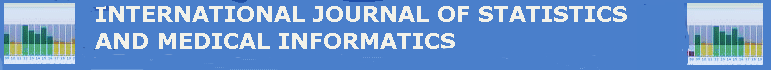### Application of Quantile regression in clinical research: An overview with the help of R and SAS statistical package

Editor IJSMI

#### Abstract

Normally the relationship between two variables x and y is studied using the linear regression equation. Linear regression equation requires normality and homoscedasticity (equal variance) assumption. When the normality and homoscedasticity assumptions are violated the linear regression estimates are not valid. Quantile regression method overcomes the drawbacks of Linear Regression and can be applied when the data is skewed and equal variance assumptions are violated. This paper provides an overview of application of quantile regression in the clinical research using R and SAS statistical package.

#### Keywords

Quantile Regression; Linear Regression; SAS; R package

PDF

#### References

Seber, George AF, and Alan J. Lee. Linear regression analysis. Vol. 936. John Wiley & Sons, 2012.

Koenker, R. (2005). Quantile regression (No. 38). Cambridge university press.

Koenker, R. (2017). Quantile Regression: 40 Years On. Annual Review of Economics, 9(1).

Yu, K., Lu, Z., & Stander, J. (2003). Quantile regression: applications and current research areas. Journal of the Royal Statistical Society: Series D (The Statistician), 52(3), 331-350.

Beyerlein, A. (2014). Quantile regression—opportunities and challenges from a user's perspective. American journal of epidemiology, 180(3), 330-331.

Lê Cook, B., & Manning, W. G. (2013). Thinking beyond the mean: a practical guide for using quantile regression methods for health services research. Shanghai archives of psychiatry, 25(1), 55.

Austin, P. C., Tu, J. V., Daly, P. A., & Alter, D. A. (2005). The use of quantile regression in health care research: a case study examining gender differences in the timeliness of thrombolytic therapy. Statistics in medicine, 24(5), 791-816.

Hosmer, D.W., Lemeshow, S. and Sturdivant, R.X. (2013) Applied Logistic Regression: Third Edition.

https://support.sas.com/documentation/cdl/en/statug/63033/HTML/default/viewer.htm#statug_qreg_sect008.htm (Accessed on 25-Mar-2017)

https://cran.r-project.org/web/packages/quantreg/index.html (Accessed on 25-Mar-2017)

KOENKER, R. QUANTILE REGRESSION IN R: A VIGNETTE.

Chen, J., Vargas‐Bustamante, A., Mortensen, K., & Thomas, S. B. (2014). Using quantile regression to examine health care expenditures during the great recession. Health services research, 49(2), 705-730.

Peng, L., & Huang, Y. (2008). Survival analysis with quantile regression models. Journal of the American Statistical Association, 103(482), 637-649

Yin, G., & Cai, J. (2005). Quantile regression models with multivariate failure time data. Biometrics, 61(1), 151-161.

Lin, G., & Rodriguez, R. N. (2013). Using the QUANTLIFE Procedure for Survival Analysis. In Proceedings of the SAS Global Forum 2013 Conference, Cary, NC: SAS Institute Inc. Available at http:/support. sas. com/resources/papers/proceedings11/421-2013. pdf.

SAS and all other SAS Institute Inc. product or service names are registered trademarks or trademarks of SAS Institute Inc. in the USA and other countries. ® indicates USA registration.

DOI: http://dx.doi.org/10.3000/ijsmi.v2i1.5